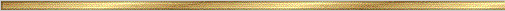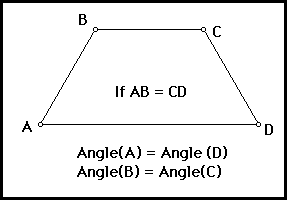# Isosceles Trapezoid Conjecture### Explanation:

A trapezoid is a quadrilateral with exactly one pair of parallel sides. In a trapezoid the parallel sides are called bases. A pair of angles that share a base as a common side are called a pair of base angles. A trapezoid with the two non-parallel sides the same length is called an isosceles trapezoid. This conjecture tells us that the base angles of an isosceles trapezoid are equal in measure.

### The precise statement of the conjecture is:

Conjecture (Isosceles Trapezoid ): The base angles of an isosceles trapezoid are equal in measure.